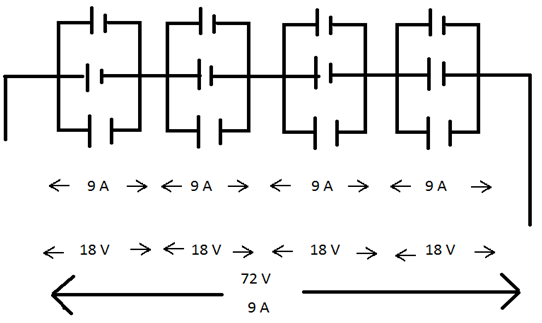Draw neat and labelled diagrams. One solar panel produces a potential difference of 18 V and current of 3A. Describe how you can obtain a potential difference of 72 Volts and current of 9 A with a solar array using solar panels. You can use sign of a battery for a solar panel.

Question 24.

Draw neat and labelled diagrams.

One solar panel produces a potential difference of 18 V and current of 3A. Describe how you can obtain a potential difference of 72 Volts and current of 9 A with a solar array using solar panels. You can use sign of a battery for a solar panel.Series connection of solar cells: The potential difference obtained from this combination is addition of the potential differences of individual solar cells. However, the current generated from this combination is equal to the current from an individual cell.

Parallel connection of solar cells: If two solar cells are connected in parallel, the current generated from this combination is the summation of the currents from an individual solar cells. However, the potential difference obtained from this combination is the same as the potential difference obtained from individual cell.

Thus, the current produced in each loop is equal to 9A.

The current flowing through the circuit is 9A as the 4 loops are connected in series with each other.

The voltage produced is 18V across each loop, as the voltage remains constant in parallel connection.

The total voltage is 72V across the entire combination, as the potential difference gets added in series connection.